# How to make formulas in Excel

The tutorial explains how to write formulas in Excel, beginning with very simple ones. You will learn how to create a formula in Excel using constants, cell references and defined names. Also, you will see how to make formulas using the function wizard or enter a function directly in a cell.

In the previous article we've started to explore a fascinating word of Microsoft Excel formulas. Why fascinating? Because Excel provides formulas for almost anything. So, whatever problem or a challenge you are facing, chances are that it can be solved by using a formula. You just need to know how to make a proper one :) And it is exactly what we are going to discuss in this tutorial.

For starters, any Excel formula begins with the equal sign (=). So, whatever formula you are going to write, begin by typing = either in the destination cell or in the Excel formula bar. And now, let's have a closer look at how you can make different formulas in Excel.

## How to make a simple Excel formula by using constants and operators

In Microsoft Excel formulas, constants are numbers, dates or text values that you enter directly in a formula. To create a simple Excel formula using constants, just do the following:

• Select a cell where you want to output the result.
• Type the equal symbol (=), and then type the equation you want to calculate.
• Press the Enter key to complete your formula. Done!

Here is an example of a simple subtraction formula in Excel:

`=100-50`

## How to write formulas in Excel using cell references

Instead of entering values directly in your Excel formula, you can refer to the cells, containing those values.

For example, if you want to subtract a value in cell B2 from the value in cell A2, you write the following subtraction formula: `=A2-B2`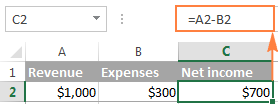When making such a formula, you can type the cell references directly in the formula, or click the cell and Excel will insert a corresponding cell reference in your formula. To add range reference, select the range of cells in the sheet.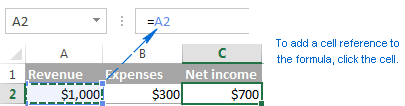Note. By default, Excel adds relative cell references. To switch to another reference type, press the F4 key.

A big advantage of using cell references in Excel formulas is that whenever you change a value in the referred cell, the formula recalculates automatically without you having to manually update all the calculations and formulas on your spreadsheet.

## How to create an Excel formula by using defined names

To take a step further, you can create a name for a certain cell or a range of cells, and then refer to that cell(s) in your Excel formulas by simply typing the name.

The fastest way to create a name in Excel, is to select a cell(s) and type the name directly in the Name Box. For example, this is how you create a name for cell A2: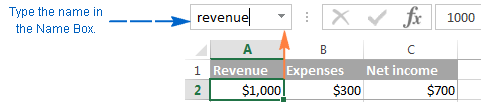A professional-like way to define a name is via the Formulas tab > Defined names group or Ctrl+F3 shortcut. For the details steps, please see creating a defined name in Excel.

In this example, I've created the following 2 names:

• revenue for cell A2
• expenses for cell B2

And now, to calculate the net income, you can type the following formula in any cell on any sheet within the workbook in which those names were created: `=revenue-expenses`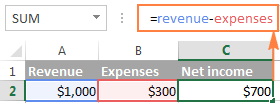In the same manner, you can use names instead of cell or range references in arguments of Excel functions.

For example, if you create the name 2015_sales for cells A2:A100, you can find a total of those cells by using the following SUM formula: `=SUM(2015_sales)`

Of course, you can get the same result by supplying the range to the SUM function: `=SUM(A2:A100)`

However, defined names make Excel formulas more understandable. Also, they can significantly expedite creating formulas in Excel especially when you are using the same range of cells in multiple formulas. Instead of navigating between different spreadsheets to find and select the range, you just type its name directly in the formula.

## How to make Excel formulas by using functions

Excel functions are nothing else than predefined formulas that perform the required calculations behind the scene.

Each formula begins with an equal sign (=), followed by the function name and the function arguments entered within the parentheses. Each function has specific arguments and syntax (particular order of arguments).

For more information, please see a list of the most popular Excel functions with formula examples and screenshots.

In your Excel spreadsheets, you can create a function-based formula in 2 ways:

### Create a formula in Excel by using the Function Wizard

If you do not feel very comfortable with Excel spreadsheet formulas yet, the Insert Function wizard will give you a helpful hand.

#### 1. Run the function wizard.

To run the wizard, click the Insert Function button on the Formulas tab > Function Library group, or pick a function from one of the categories: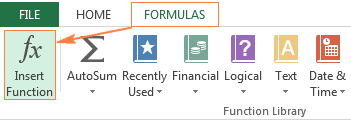Alternatively, you can click the Insert Function button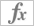to the left of the formula bar.

Or, type the equal sign (=) in a cell and pick a function from the drop-down menu to the left of the formula bar. By default, the drop-down menu displays 10 most recently used functions, to get to the full list, click More Functions...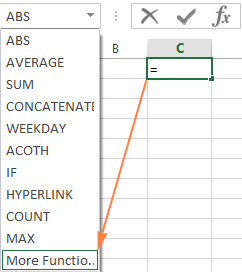#### 2. Find the function you want to use.

When the Insert Function wizard appears, you do the following:

• If you know the function name, type it in the Search for a function field and click Go.
• If you are not sure exactly what function you need to use, type a very brief description of the task you want to solve in the Search for a function field, and click Go. For example, you can type something like this: "sum cells", or "count empty cells".
• If you know what category the function belongs to, click the small black arrow next to Select a category and choose one of the 13 categories listed there. The functions belonging to the selected category will appear in the Select a function

You can read a short description of the selected function right under the Select a function box. If you need further details regarding that function, click the Help on this function link near the bottom of the dialog box.

Once you've found the function you wish to use, select it and click OK.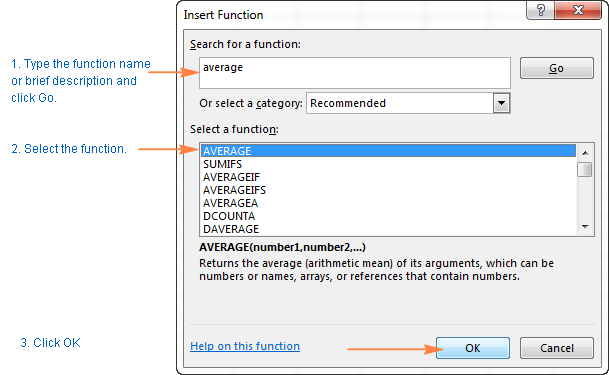#### 3. Specify the function arguments.

In the second step of the Excel function wizard, you are to specify the function's arguments. Good news is that no knowledge of the function's syntax is required. You just enter the cell or range references in the arguments' boxes and the wizard will take care of the rest.

To enter an argument, you can either type a cell reference or range directly into the box. Alternatively, click the range selection icon next to the argument (or simply put the cursor into the argument's box), and then select a cell or a range of cells in the worksheet using the mouse. While doing this, the function wizard will shrink to a narrow range selection window. When you release the mouse button, the dialog box will be restored to its full size.

A short explanation for the currently selected argument is displayed right under the function's description. For more details, click the Help on this function link near the bottom.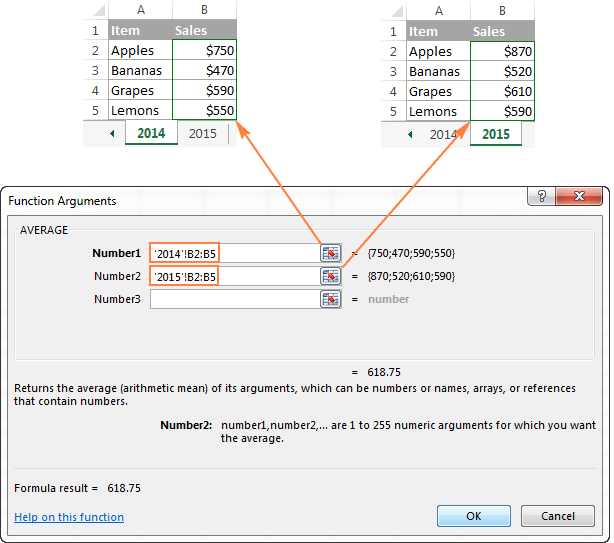Excel functions allow you to perform calculations with cell residing on the same worksheet, different sheets and even different workbooks. In this example, we are calculating the average of sales for 2014 and 2015 years located in two different spreadsheets, which in why the range references in the above screenshot include the sheet names. Find more about how to reference another sheet or workbook in Excel.

As soon as you've specified an argument, the value or array of values in the selected cell(s) will be displayed right to the argument's box.

#### 4. Complete the formula.

When you have specified all the arguments, click the OK button (or just press the Enter key), and the completed formula is entered into the cell.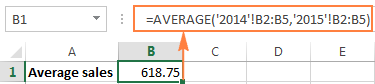### Write a formula directly in a cell or formula bar

As you've just seen, creating a formula in Excel by using the function wizard is easy, thought it's quite a long multi-step process. When you have some experience with Excel formulas, you might like a faster way - typing a function directly into a cell or formula bar.

As usual, you start by typing the equal sign (=) followed by the function name. As you do this, Excel will perform some kind of incremental search and display a list of functions that match the part of the function's name you've already typed: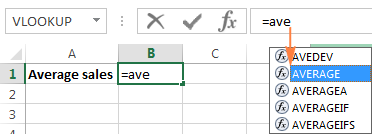So, you can either finish typing the function name on your own or select from the displayed list. Either way, as soon as you type an opening parenthesis, Excel will show the function screen tip highlighting the argument you need to enter next. You can type the argument in the formula manually, or click a cell (select a range) in the sheet and have a corresponding cell or range reference added to the argument.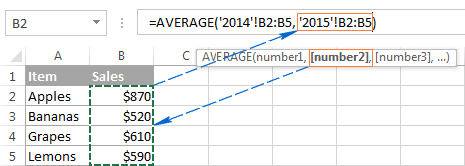After you've input the last argument, type the closing parenthesis and hit Enter to complete the formula.

Tip. If you are not quite familiar with the function's syntax, click the function name and the Excel Help topic will pop-up right away.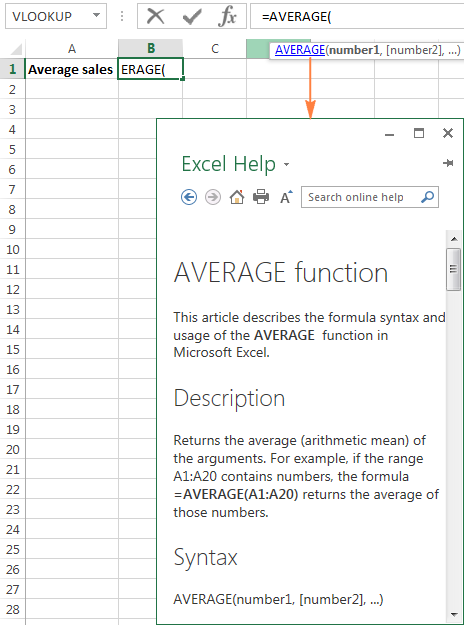This is how you create formulas in Excel. Nothing difficult at all, is it? In the next few articles, we will continue our journey in the intriguing realm of Microsoft Excel formulas, but those are going to be short tips to make your work with Excel formulas more efficient and productive. Please stay tuned!

## You may also be interested in

1. if i insert some data in A1 cell today, and that data will automatically display on another A3 cell and next day i insert some data in A1 cell in this place i need to display in cell A3 Both First Day Data and Second Day Data it self , Kinddly Suggest Some Idea

• Hi!
A cell can contain only one value. Or use a VBA macro to combine the old and new values of an A3 cell into one text string.

2. Hi, i have a question. The above post says about the existing formulas in Excel.
In Excel 2021, there is custom formula option using java script and saving the file.
Is there any way to insert custom formula in MS Excel 2016.
For example, I require numbers to be presented in Text as well

Cell A1: 2000
Cell A2: Two Thousand

There is option for the same in 2021 version of MS Excel "=Spellnumber" but, how to do the same in 2016 version, without VBA and macro enabled workbook, to be specific

3. Hi kindly help me I intent calculating an undisclosed turnover in other to arrive at the right tax by using a formula from the excel

4. 45/80+34/40+23/80 which formula in used in excel
Average in same and percentage

5. I need a formula,

Eg

Customer name Achieve QTY Basic value
A 5 150
B 5 150
A 5 150
C 15 450
B 5 150
A 5 150

this is the details here i need to take customer name "A" first "10" basic value.
Result
A 5+5 150+150

A 10 300
like this
any one can help me.

• Hello!
Sorry, it's not quite clear what you are trying to achieve. Customer A is recorded 3 times, but the result is counted in two. How many values do you need to calculate?

• Hello, if you haven't yet got answer for your question... Use a =SUMIF formula. It gives you options for the above.

6. An amount of 1000 need to be increased by 500 every 3 yrs. from 01.04.2021 till 31.12.2026. Kindly frame a formula

7. wthat is the formula of 120-68.55% in excel or k9-19%

8. HI! GOOD DAY
I NEED A TO CALCULATE POSITION IN EXCEL. THANK YOU.

9. Hello, i am working on an excel sheet i need help
I want to know who are taking medicine in a village
I fill a table column by the name of the villagers and another by medicine and the 3rd is insurence
The medicine and indusrence column only include yes or no
How could i make on another page only the name of the villager display automatically if he takes medicine and doesn't have insurence?

10. column-A , column-B
1
2
3
4
5
6
7
8
9
10
11
12
13
14
15........
and serial go more then 4000,
so i want a print out on paper size A4, like this:-
column-A , column-B , column-C, column-D
1 , 11 , 21 , 31
2 , 12 , 22 , 32
3 , 13 , 23 , 33
4 , 14 , 24 , 34
5 , 15 , 25 , 35
6 , 16 , 26 , 36
7 , 17 , 27 , 37
8 , 18 , 28 , 38
9 , 19 , 29 , 39
10 , 20 , 30 , 40 and so on